# The i-j System

The i-j System

Consider the following figure, which shows a point P with coordinates $$\left( {3,4} \right)$$ on a rectangular coordinate system with origin O: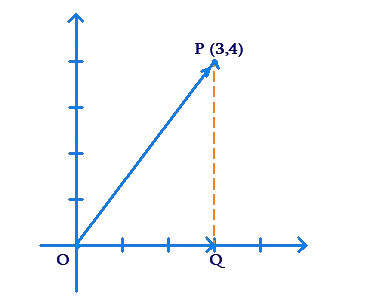How can we completely specify the vector $$\overrightarrow {OP}$$ in a mathematical sense? Note that to reach P from O, we can first move along the vector $$\overrightarrow {OQ}$$, and then along the vector $$\overrightarrow {QP}$$. Using the triangle law, we can write the following relation:

$\overrightarrow {OP} = \overrightarrow {OQ} + \overrightarrow {QP}$

Now, $$\overrightarrow {OQ}$$ is a vector 3 units long, and points in the positive x-direction. Let us denote a unit vector in the positive x-direction by $$\hat i$$. Then, we can write $$\overrightarrow {OQ}$$ as $$3\hat i$$.

Similarly, we observe that $$\overrightarrow {QP}$$ is a vector 4 units long, and points in the positive y-direction. If we denote a unit vector in the positive y-direction by $$\hat j$$, we can write $$\overrightarrow {QP}$$ as $$4\hat j$$.

These observations are included in the following figure: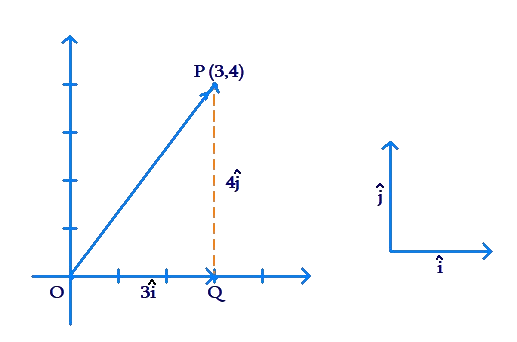Thus, we can write $$\overrightarrow {OP}$$ in terms of $$\hat i$$ and $$\hat j$$ as follows:

$\overrightarrow {OP} = \overrightarrow {OQ} + \overrightarrow {QP} = 3\hat i + 4\hat j$

Now, $$\overrightarrow {OP}$$ is completely specified in a mathematical sense, since this representation tells us exactly how to reach P from O: first move 3 units along the positive x-direction ( $$3\hat i$$), and then move 4 units parallel to the positive y-direction ( $$4\hat j$$).

The following figure shows a point A with coordinates $$\left( { - 4,1} \right)$$: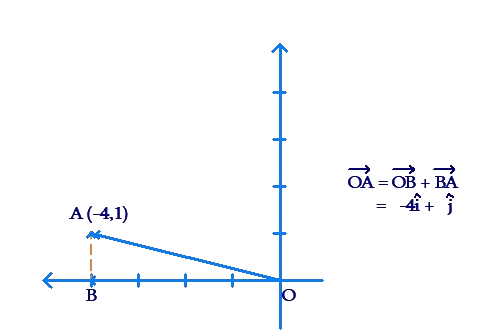In terms of $$\hat i$$ and $$\hat j$$, we can write the vector $$\overrightarrow {OA}$$ as follows:

$\overrightarrow {OA} = \overrightarrow {OB} + \overrightarrow {BA} = - 4\hat i + \hat j$

Consider the following figure, which shows a right-angled triangle ABC with the specified coordinates: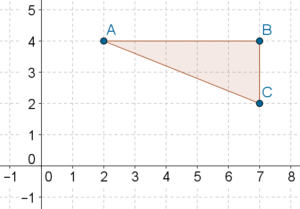Note that vector  is 5 units long, and points in the positive x-direction. Thus, we can write $$\overrightarrow {AB} = 5\hat i$$. Vector $$\overrightarrow {BC}$$ points in the negative y-direction and is 2 units long, so we can write $$\overrightarrow {BC} = - 2\hat j$$. Using the triangle law, we have:

$\overrightarrow {AC} = \overrightarrow {AB} + \overrightarrow {BC} = 4\hat i - 2\hat j$

Example 1: Observe the following figure, which shows three vectors $$\overrightarrow a$$, $$\overrightarrow b$$ and $$\overrightarrow c$$: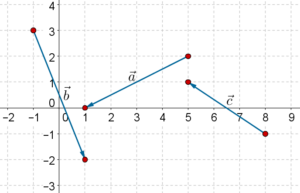Specify these vectors in terms of $$\hat i$$ and $$\hat j$$.

Solution: To move from the starting point of vector $$\overrightarrow a$$ to its tip, we need to move 4 units in the negative x-direction, and 2 units in the negative y-direction: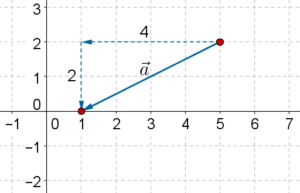Thus,

$\overrightarrow a = - 4\hat i - 2\hat j$

Following a similar approach, we have:

$\begin{array}{l}\overrightarrow b = 2\hat i - 5\hat j\\\overrightarrow c = - 3\hat i + 2\hat j\end{array}$